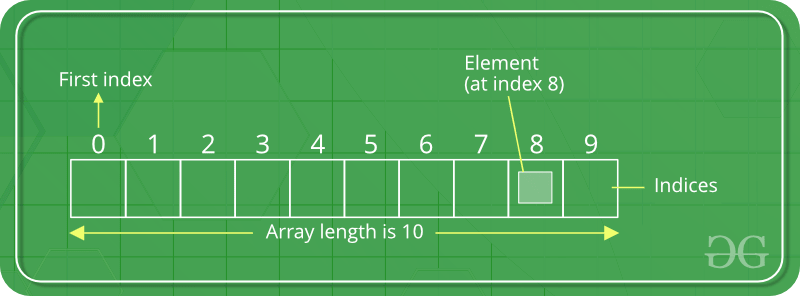Open In App

# Dart Programming – List

In Dart programming, List data type is similar to arrays in other programming languages. List is used to representing a collection of objects. It is an ordered group of objects. The core libraries in Dart are responsible for the existence of the List class, its creation, and manipulation.

### Logical Representation of ListThe index of the element represents the position of the specific data and when the list item of that index is called the element is displayed. Generally, the list item is called from its index.

### Types of List

There are broadly two types of lists on the basis of their length:

1. Fixed Length List
2. Growable List

### Fixed Length List

Here, the size of the list is declared initially and can’t be changed during runtime.

Syntax:

`List ? list_Name = List.filled(number of elements, E, growanle:boolean);`

Example:

## Dart

 `void` `main()``{``    ``List? gfg = List.filled(5, null, growable: ``false``);``    ``gfg = ``'Geeks'``;``    ``gfg = ``'For'``;``    ``gfg = ``'Geeks'``;` `    ``// Printing all the values in List``    ``print(gfg);` `    ``// Printing value at specific position``    ``print(gfg);``}`

Output:

```[Geeks, For, Geeks, null, null]
Geeks```

### Growable List

This type of list is declared without declaring the size of the list. Its length can be changed during runtime.

Adding a value to the growable list:

## Dart

 `void` `main()``{``    ``var gfg = [ ``'Geeks'``, ``'For'` `];` `    ``// Printing all the values in List``    ``print(gfg);` `    ``// Adding new value in List and printing it``    ``gfg.add(``'Geeks'``); ``// list_name.add(value);``    ``print(gfg);``}`

Output:

```[Geeks, For]
[Geeks, For, Geeks]```

Adding multiple values to the growable list:

## Dart

 `void` `main()``{``    ``var gfg = [ ``'Geeks'` `];` `    ``// Printing all the values in List``    ``print(gfg);` `    ``// Adding multiple values in List and printing it` `    ``// list_name.addAll([val 1, val 2, ...]);``    ``gfg.addAll([ ``'For'``, ``'Geeks'` `]);``    ``print(gfg);``}`

Output:

```[Geeks]
[Geeks, For, Geeks]```

Adding a value to the growable list at a specific index:

## Dart

 `void` `main()``{``    ``var gfg = [ ``'Geeks'``, ``'Geeks'` `];` `    ``// Printing all the values in List``    ``print(gfg);` `    ``// Adding new value in List at``    ``// specific index and printing it` `    ``// list_name.insert(index, value);``    ``gfg.insert(1, ``'For'``);``    ``print(gfg);``}`

Output:

```[Geeks, Geeks]
[Geeks, For, Geeks]```

Adding multiple values to the growable list at specific indexes:

## Dart

 `void` `main()``{``    ``var gfg = [ ``'Geeks'` `];` `    ``// Printing all the values in List``    ``print(gfg);` `    ``// Adding new value in List at``    ``// specific index and printing it` `    ``// list_name.insertAll(index, list_of_values);``    ``gfg.insertAll(1, [ ``'For'``, ``'Geeks'` `]);``    ``print(gfg);` `    ``// Element at index 1 in list``    ``print(gfg);``}`

Output:

```[Geeks]
[Geeks, For, Geeks]
For```

### Types of List (Basis of its Dimensions)

There are various numbers on the list based on dimension, but the most popular among them are:

1. 1-Dimensional (1-D) List
2. 2-Dimensional (2-D) List
3. 3-Dimensional (3-D) List
4. Multidimensional List

Here, we have already discussed the 1-D list.

### 2-Dimensional (2-D) List

Here, the list is defined in two dimensions and thus forming the look of the table.

Creating 2-D List:

## Dart

 `void` `main()``{``    ``int` `a = 3;``    ``int` `b = 3;` `    ``// Creating two dimensional list``    ``var gfg = List.generate(a, (i) = > List(b), growable: ``false``);` `    ``// Printing its value``    ``print(gfg);` `    ``// Inserting values``    ``for` `(``int` `i = 0; i < 3; ++i) {``        ``for` `(``int` `j = 0; j < 3; ++j) {``            ``gfg[i][j] = i + j;``        ``}``    ``}``    ``// Printing its value``    ``print(gfg);``}`

Output:

```[[null, null, null], [null, null, null], [null, null, null]]
[[0, 1, 2], [1, 2, 3], [2, 3, 4]]```

Another way of creating a 2-D List:

## Dart

 `void` `main()``{``    ``// Creating three dimensional list``    ``var gfg = List.generate(3, (i) = > List.generate(3, (j) = > i + j));` `    ``// Printing its value``    ``print(gfg);``}`

Output:

`[[0, 1, 2], [1, 2, 3], [2, 3, 4]]`

There is also another way of creating a 2-D list, i.e giving the values associated with the indexes and it will lead to the creation of the 2-D list.

### 3-Dimensional (3-D) List

The representation of a 3-D list is quite difficult but its creation is similar to that of a 2-D list.

Example:

## Dart

 `void` `main()``{``    ``// Creating three dimensional list``    ``var gfg = List.generate(3, (i) = > List.generate(3,``                               ``(j) = > List.generate(3,``                               ``(k) = > i + j + k)));` `    ``// Printing its value``    ``print(gfg);``}`

Output:

`[[[0, 1, 2], [1, 2, 3], [2, 3, 4]], [[1, 2, 3], [2, 3, 4], [3, 4, 5]], [[2, 3, 4], [3, 4, 5], [4, 5, 6]]]`

Note: In a similar fashion one can create an n-dimensional List i.e by using the “List.generate()” method.